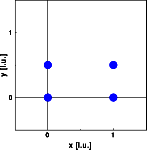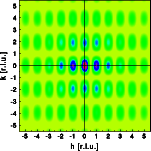Interactive Tutorial about Diffraction Reciprocal space III: Rectangular latticeReciprocal space Reciprocity Square lattice Rectangular lattice Oblique lattice Translation of lattice Rotation lattice Interactive examples 2D crystal builder Planes and HKL's Finite size effect Goto Contents In this example an orthorhombic lattice is simulated. The vertical unit cell length is half the horizontal unit cell length. Two by two unit cells are simulated, each containing one atom at (0 0). This miniature crystal is sufficient in size to illustrate the geometrical relationships. By increasing the size, we will just decrease the FWHM of the Bragg reflections. The units in the pictures are given in unit cell units of the squared lattice in the first example. The pictures below show the atoms and the corresponding Fourier transform. Click on the pictures to get a full screen version.The reciprocal space geometry corresponds to that of the real space. The reciprocal base vectors are orthogonal to each other, but the vertical reciprocal base vector is twice as long as the horizontal. © Th. Proffen and R.B. Neder, 2003